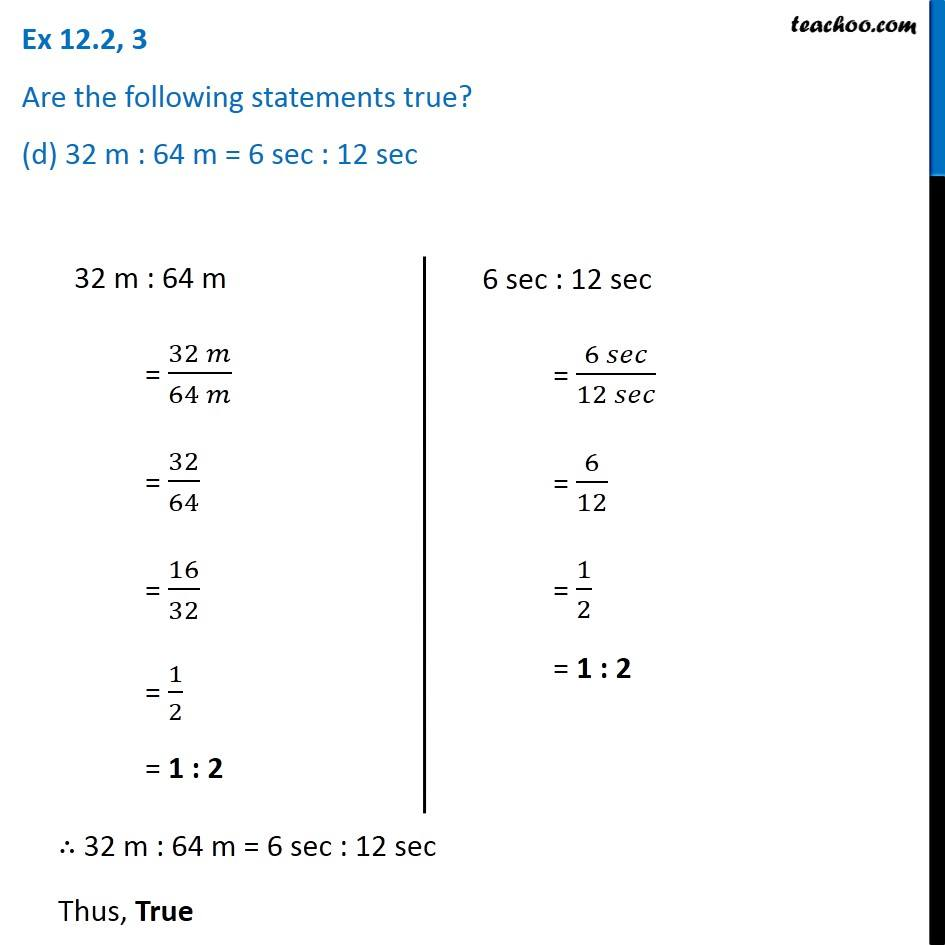Ex 12.2

Chapter 12 Class 6 Ratio and Proportion
Serial order wiseLearn in your speed, with individual attention - Teachoo Maths 1-on-1 Class

### Transcript

Ex 12.2, 3 Are the following statements true? (d) 32 m : 64 m = 6 sec : 12 sec 6 sec : 12 sec = (6 𝑠𝑒𝑐)/(12 𝑠𝑒𝑐) = 6/12 = 1/2 = 1 : 2 ∴ 32 m : 64 m = 6 sec : 12 sec Thus, True